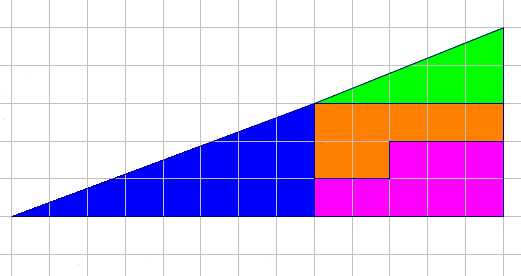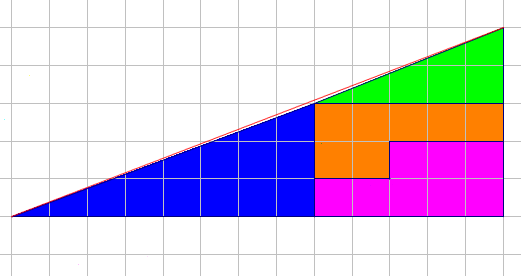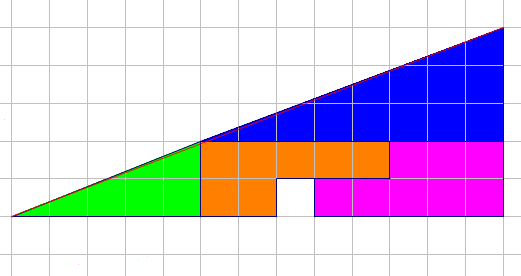You a can also see the puzzle. According to the 'Missing square puzzle' Wikipedia Page the puzzle was created by Paul Curry, an amateur magician.The areas of the four sections are:

• Blue = ½ x 8 x 3 = 12
• Green = ½ x 5 x 2 = 5
• Orange = 7
• Pink = 8

A total of 12 + 5 + 7 + 8 = 32 squares.

However, the area of the overall triangle appears to be

• ½ x 13 x 5 = 32.5 squares

Clearly something unusual is going on.Since the triangle should have an area of 32.5 squares and the pieces only add to 32 squares, the pieces cannot form a true triangle.

What appears to be the diagonal of the larger triangle is actually bent inwards slightly. The true diagonal is drawn in red.When the pieces are moved around, again they do not form a true triangle, this time what appears to be the diagonal of the larger triangle is actually bent outwards slightly.

The difference between the first one bent inwards, and the second one bent outwards measures exactly 1 square, which is the missing square.

Note: BrainBashers has a Dark Mode setting.